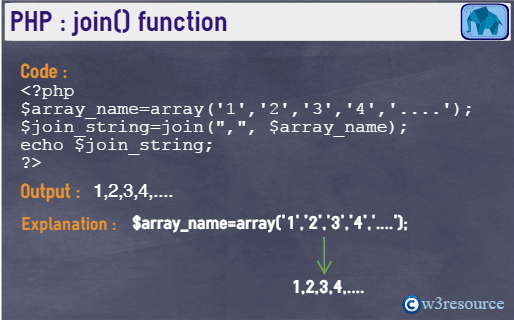# PHP: join() function

## Description

The join() function is used to join array elements with a string. The join() function is similar to implode() function.

Version:

(PHP 4 and above)

Syntax:

` join (string_join, array_name)`

Parameters:

Name Description Required /
Optional
Type
string_join String-join is work as a connector between the array elements. The default value is a empty string (" "). Optional String
array_name The array who's elements will be joined. Required Array

Return values:

A string representation of all the array elements in the same order, with the string_join string between each array element.

Value Type: String.

Pictorial PresentationExample:

``````<?php
\$array_name=array('1','2','3','4','....');
\$join_string=join(",", \$array_name);
echo \$join_string;
echo '<br>';
\$array_name=array('A','B','C','D','E');
\$join_string=join("-", \$array_name);
echo \$join_string;
?>
``````

Output:

```1,2,3,4,....
A-B-C-D-E```

View the example in the browser

PHP Function Reference

Previous: implode
Next: ltrim

﻿

## PHP: Tips of the Day

In PHP, there are two versions of logical AND and OR operators.

Operator True if
\$a and \$b Both \$a and \$b are true
\$a && \$b Both \$a and \$b are true
\$a or \$b Either \$a or \$b is true
\$a || \$b Either \$a or \$b is true

Note that the && and || opererators have higher precedence than and and or. See table below:

Evaluation Result of \$e Evaluated as
\$e = false || true True \$e = (false || true)
\$e = false or true False (\$e = false) or true

Because of this it's safer to use && and || instead of and and or.